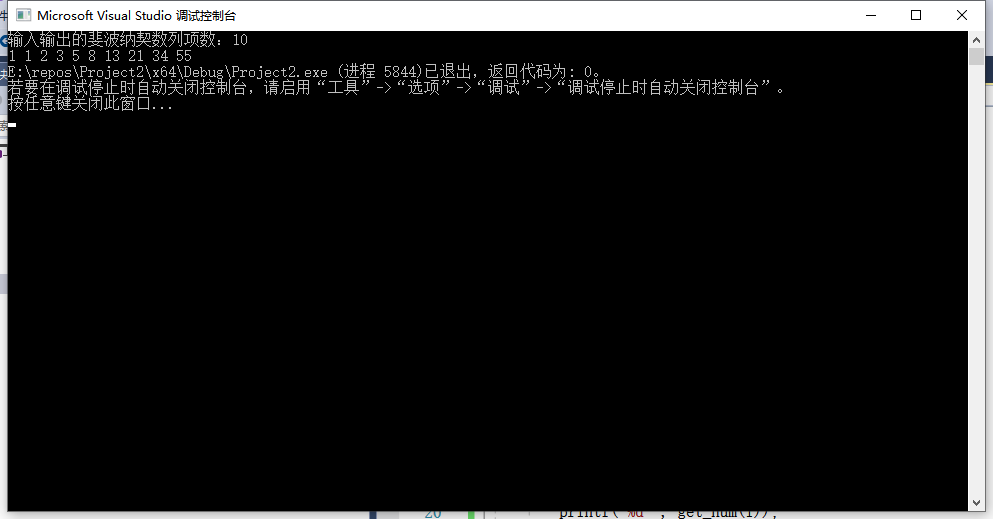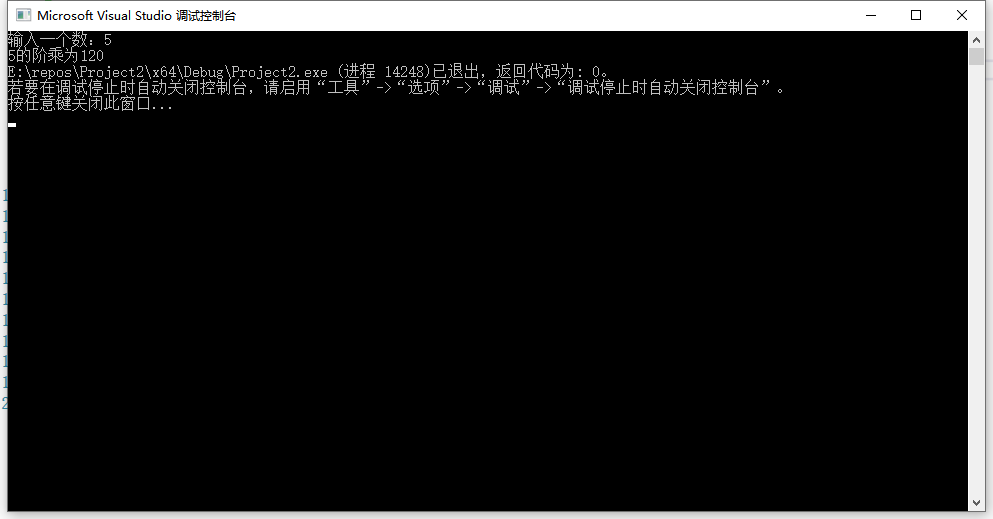• 【递归函数】递归函数求斐波那契数列前N项和（二）题目描述输入输出样例输入样例输出分析代码实现 题目描述 斐波那契数列定义：an=an-1+an-2，即第n项值等于前两项之和。 已知斐波那契数列第一、第二项值为1，1，...
【C语言】【递归函数】用递归函数求斐波那契数列前N项和（二）题目描述输入输出样例输入样例输出分析代码实现
题目描述

斐波那契数列定义：an=an-1+an-2，即第n项值等于前两项之和。
已知斐波那契数列第一、第二项值为1，1，求该数列前n项之和

输入

第一行输入m，表示有m个斐波那契数列要求和
接下去n行都输入一个整数n ，n不大于30

输出

m个斐波那契数列的和的结果，每行一个，一共m行

样例输入

3
3
4
5

样例输出

4
7
12

分析
由于问题要求输入的整数n不大于30，故可以定义一个用于求斐波那契数列前30项的函数，将求出的数据保存到一维数组中。再定义一个用于求和的函数，在此函数中直接调用已经储存到数组中的值。
代码实现
#include "stdio.h"

void fb(int a[], int p); //将数列数据存于数组中，p表示当前第p项数据
int sum(int a[], int n); //计算完数列，只要求前n想数组单元格和即可
int main()
{
int m, n, i, h;
int r = {0, 1, 1};
fb(r, 3); //从第3项开始求，直接计算3~30项的数据并放入数组中
scanf("%d", &m);

for (i = 0; i < m; i++)
{
scanf("%d", &n);
h = sum(r, n);
printf("%d\n", h);
}
}

void fb(int a[], int p)
{
if (p == 31) //计算到第30项
return;
else
{
a[p] = a[p - 1] + a[p - 2]; //计算a[p]的值
fb(a, p + 1);               //递归调用函数
}
}

int sum(int a[], int n)
{
int i, fbsum = 0;
for (i = 1; i <= n; i++)
{
fbsum = fbsum + a[i];
}
return fbsum;
}



展开全文• 例1：使用递归函数求斐波那契数列 斐波那契数列可表示为：F(1)=1，F(2)=1, F(n)=F(n - 1)+F(n - 2)（n ≥ 3，n ∈ N*） 求数列第n项可以递归函数，只有当n1和n2时函数结束；其他情况一直调用他本身。 代码： #...
编程语言中，函数Func(Type a,……)直接或间接调用函数本身，则该函数称为递归函数。递归函数不能定义为内联函数。
执行递归函数将反复调用其自身，每调用一次就进入新的一层，当最内层的函数执行完毕后，再一层一层地由里到外退出。
例1：使用递归函数求斐波那契数列
斐波那契数列可表示为：F(1)=1，F(2)=1, F(n)=F(n - 1)+F(n - 2)（n ≥ 3，n ∈ N*）
求数列第n项可以用递归函数，只有当"n=1"和"n=2"时函数结束；其他情况一直调用他本身。
代码：
#include <stdio.h>
long get_num(int n)
{
if (n == 1 || n == 2)
{
return 1;
}
else
{
return get_num(n - 1) + get_num(n - 2);
}
}
int main()
{
int n, i;
printf("输入的斐波纳契数列项数：");
scanf_s("%d", &n);
for (i = 1; i <= n; i++)
{
printf("%ld ", get_num(i));
}
return 0;
}

功能：在控制台上输入一个数，输出斐波那契数列第1项到第n项。例2：使用递归函数求阶乘
阶乘的计算方法：
大于等于1
任何大于等于1 的自然数n 阶乘表示方法：
n!= 1 * 2 * 3 * ··· * (n-1) n
或
n!=n * (n-1)!
0的阶乘
0！=1。
求阶乘可以用递归函数，只有当"n=0"和"n=1"时函数结束；其他情况一直调用他本身。
#include <stdio.h>
long get_num(int n)
{
if (n == 0 || n == 1)
{
return 1;
}
else
{
return get_num(n - 1)* n;
}
}
int main()
{
int n;
printf("输入一个数：");
scanf_s("%d", &n);
printf("%d的阶乘为%ld", n, get_num(n));
return 0;
}

功能：在控制台上输入一个数，输出该数值的阶乘。展开全文• 【递归函数】递归函数求斐波那契数列第N项值（一）题目描述输入输出样例输入样例输出分析代码实现 题目描述 已知斐波那契数列为1，1，2，3，5，8，13，7……，第一、第二项值为1，1，从第3项起，每项的值都等于前2...
【C语言】【递归函数】用递归函数求斐波那契数列第N项值（一）题目描述输入输出样例输入样例输出分析代码实现
题目描述
已知斐波那契数列为1，1，2，3，5，8，13，7……，第一、第二项值为1，1，从第3项起，每项的值都等于前2项的和，求该数列第n项的值。
输入
一个整数n
输出
第n项的数据
样例输入
4
样例输出
3
分析
定义一个用于求第n项的函数。
当输入的整数n=1或2时，可以直接输出1；当输入的整数n>=3,利用函数递归求出前两项，进行求和。
代码实现
#include"stdio.h"
int fb(int n);  //斐波那契求和函数原型申明
int main()
{
int n,s;
scanf("%d",&n);
s=fb(n);
printf("%d\n",s);
}

int fb(int n)
{
int r;
if(  n==1 || n==2)
r=  1;
else
r=  fb(n-1)+fb(n-2);
return r;
}



展开全文• 斐波那契数列：");  scanf("%d",&n);  //int *a = (int *)malloc((n+1)*sizeof(int));//如需存储，使用动态内存分配n+1个空间进行存储  int i;...有问必答
• 【问题描述】编写函数f，功能是递归的方法求斐波那契数列的第n项，函数原型为 int f(int n),在主函数中输入一个正整数n，调用函数f求出斐波那契数列的第n项，并在主函数中输出。 斐波那契数列：1,1,2,3,5,8,13,21...
【问题描述】编写函数f，功能是用递归的方法求斐波那契数列的第n项，函数原型为  int f(int n),在主函数中输入一个正整数n，调用函数f求出斐波那契数列的第n项，并在主函数中输出。
斐波那契数列：1,1,2,3,5,8,13,21……
【输入形式】3
【输出形式】2
【样例输入】6
【样例输出】8
源代码如下：
#include <stdio.h>
int fei(int n)
{
if(n==0)return 0;
if(n==1)return 1;
if(n>=2)return fei(n-1)+fei(n-2);
}
int main(int argc, char *argv[])
{
int a,b;
scanf("%d",&a);
b=fei(a);
printf("%d",b);
return 0;
}



展开全文• 函数调用时递归函数来实现此功能。 代码如下： #include&lt;stdio.h&gt; int fun(int n) {  if(1==n)  {  return 1;  }  if(0==n)  {  return 1;  }  return fun(n-1)+fun(n-2); } int ...
• 要求： 编写程序，将斐波那契数列前20项写入文件fab.txt中，并将斐波那契...// 求斐波那契数列 int fun(int n){ int result; if(n==1) result=1; else if(n==2) result=1; else result = fun(n-1)+fun(n-2); ret
• =2) 递归第n项。 输入格式: 输入一个正整数n（1<=n<=20） 输出格式: 输出斐波那契数列的第n项 函数接口定义： 在这里描述函数接口。例如： int cn(int n)； 在这里解释接口参数。例如：其中 n是用户传入的...
• 题目：定义Fibonacci数列如下：   / 0 n=0 f(n) 1 n=1 ...分析：在很多C语言教科书中讲到递归函数的时候，都会Fibonacci作为例子。 最快当然是矩阵快速幂了 {fn，fn-1} * {1,1数据结构
• 题目1描述：  写一个函数，输入n，求斐波那契数列的第n项。斐波那契数列的定义如下： f(n) = 0 (n = 0);... 在大多数的C语言教科书中，一般会递归求斐波那契数列。代码如下： long long Fibonacci
•  写一个函数，输入n，求斐波那契数列（Fibonacci）数列的第 n 项。斐波那契数列的定义如下：   方法1：效率很低效的解法，挑剔的面试官不会喜欢 很多C语言的教科书在讲述递归函数的时候，都会拿 Fibonacci ...剑指offer
• 斐波那契数列的定义如下：　解题思路 在学C语言的时候课本上就有斐波那契数列，实现的方法是最直观的递归，主要代码如下：long long Fabonacci(const int&amp; num) { if (num == 1) return 1; if (num =...动态规划 递归
• 写一个函数，输入 n ，求斐波那契（Fibonacci）数列的第 n 项。斐波那契数列的定义如下： F(0) = 0, F(1) = 1 F(N) = F(N - 1) + F(N - 2), 其中 N > 1. 斐波那契数列由 0 和 1 开始，之后的斐波那契数就是由之前...算法 python leetcode 数据结构
• 题目一：写一个函数，输入n，求斐波那契数列的第n项。斐波那契数列的定义如下： 1、效率很低效的解法，挑剔的面试官不会喜欢 很多C语言的教科书在讲述递归函数的时候，都户拿Fibonacci作为例子，因此很多的应聘者对...
• 解析：一直以来很多C语言教科书在讲递归函数的时候总会拿斐波那契数列作为例子。但是这不意味这Fibonacci的最合适的解法就是递归来实现。如果面试问道这题然后递归解，那基本就GG了。因为递归调用过程中有很多...C++ 算法 剑指Offer 递归 面试题
• 题目一：写一个函数，输入n，求斐波那契数列的第n项。斐波那契数列的定义如下： 1、效率很低效的解法，挑剔的面试官不会喜欢 很多C语言的教科书在讲述递归函数的时候，都户拿Fibonacci作为例子，因此很...
• 题目一：写一个函数，输入n，求斐波那契数列的第n项。斐波那契数列的定义如下： 1、效率很低效的解法，挑剔的面试官不会喜欢 很多C语言的教科书在讲述递归函数的时候，都会拿Fibonacci作为例子，因此很多的应聘者对...算法
• 题目：定义Fibonacci数列如下：  / 0 n=0 f(n)= 1 n=1  \ f(n-1)+f(n-2) n=2 输入n，最快的方法数列的第n项。...分析：在很多C语言教科书中讲到递归函数的时候，都会Fibonacci作为例子。因
• 一看到这个题目最先想到的解法就是递归，因为我们在学习c语言的时候教材在讲解递归这一知识的时候就是的这个例子，虽然经常这个问题来讲解递归函数，但是这不代表递归的解法最适合这道题目，一般在面试的时候...python 剑指offer
• 如：1、1、2、3、5…求斐波那契数列的第n项。 实现方法：采用递归函数的方式，以此算出每项，求出所求项 注意斐波那契数列此方式虽然简便，但是当项数很大时往往会运行超时 这时候就应该使用数组+while循环的办法...
• 目录 斐波那契数是什么? 斐波那契相关数学问题: 楼梯问题: 硬币问题: ...斐波那契数列（Fibonacci sequence），又称黄金分割数列、因数学家列昂纳多·斐波那契（Leonardoda Fibonacci）以兔子繁殖...
• C语言函数+Visual Studio简单的使用教程写在前面函数概述函数的调用嵌套调用递归调用递归实现循环作用递归求阶乘递归法求斐波那契数列的第n项递归法求两个正整数的最大公约数递归算法解决爬楼梯问题数组作为函数...
• 1.1+1/2+1/3+1/4+…1/10 之和 #include<stdio.h> int main(){ int i; double all; for(i=1;... //因为1和i都是int时,答案会按照整数来算，所以要1.0 ...2.输出斐波那契数列的前20项 1 1 2 3 5 8 13 21 34 55
• 函数的递归函数递归：例题：递归求 年龄例题：递归求解例题：递归求斐波那契数列函数的引用传递数组元素作为实参例题：局部变量和全局变量局部变量全局变量变量的存储方式auto{自动变量}static{静态变量}register{...
• 1）斐波那契函数 题目描述： 定义Fibonacci数列如下：  f(n) = 0 n=0 f(n) = 1 n=1,2 ...输入n，最快的方法数列的第n项。...分析：在很多C语言教科书中讲到递归函数的时候，都会F
• 相信如果一个人让我们求一个斐波那契数列，如果你学过c语言，你一定会说递归法啊，很容易就实现了，但是如果人家让你求斐波那契的第50个数，而且你对递归了解的话，估计帮你不会说递归了，如果了解够深的话，其实...# c语言用函数求斐波那契数列c语言 订阅